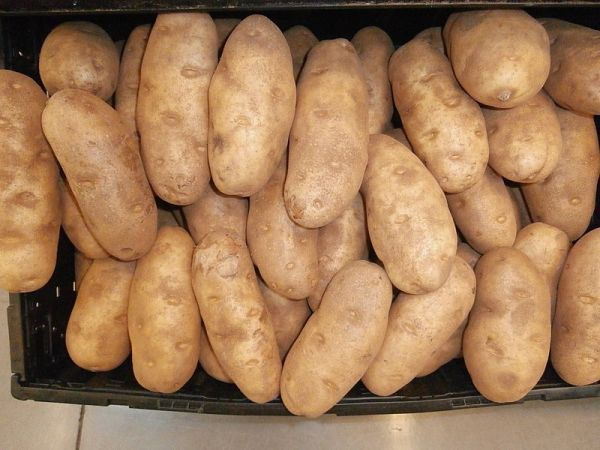# The Potato ParadoxMost of us, especially those in the food or shipping industries, know that you can save a lot of weight by dehydrating food. What if you had 100 pounds of potatoes, and each potato was 99% water. If you dehydrated those potatoes just a little, to the point that they were 98% water, how much would they weigh?

They would weigh 50 pounds. Believe it or not.

Wikipedia gives us a couple of explanations for the Potato Paradox.

Method 1

One explanation begins by saying that initially the non-water weight is 1 pound, which is 1% of 100 pounds. Then one asks: 1 pound is 2% of how many pounds? In order for that percentage to be twice as big, the total weight must be half as big.

Method 2

100 lb of potatoes, 99% water (by weight), means that there’s 99 lb of water, and 1 lb of solids. It’s a 1:99 ratio.

If the water decreases to 98%, then the solids account for 2% of the weight. The 2:98 ratio reduces to 1:49. Since the solids still weigh 1 lb, the water must weigh 49 lb for a total of 50 lbs for the answer.

You can also find the algebraic explanations on the same page, if you want to check further. By the way, potatoes are only around 79% water, but the paradox is in the math as a normal person would visualize it, not the food. Don’t confuse pounds and percentage. -via TYWKIWDBI

Source: neatorama

Rating The Potato Paradox is 5.0 / 5 Votes: 1